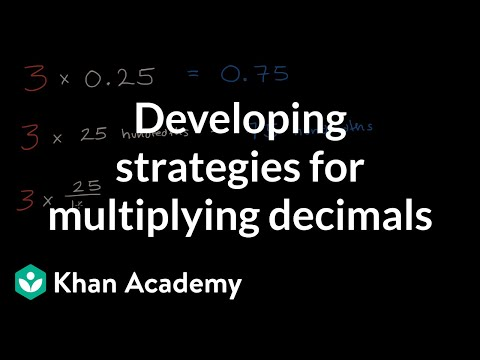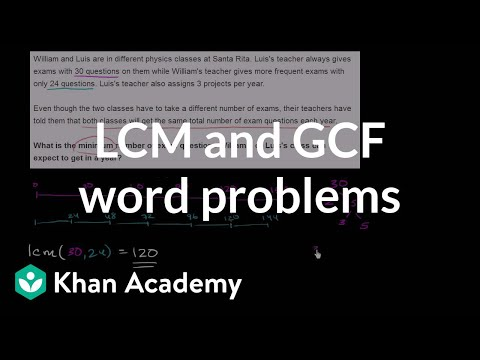# Least common multiple (Full video)

Description: Sal finds the LCM (least common multiple) of 12 and 36, and of 12 and 18. He shows how to do that using the prime factorization method, which is a just great! Created by Sal Khan. So the smallest number that is both a multiple of 36 and 12 is actually 36. What is the least common multiple of 18 and 12? The least common multiple of 18 and 12 is equal to question mark.

### Other videos you might be interested in### Developing strategies for multiplying decimals (Full video)